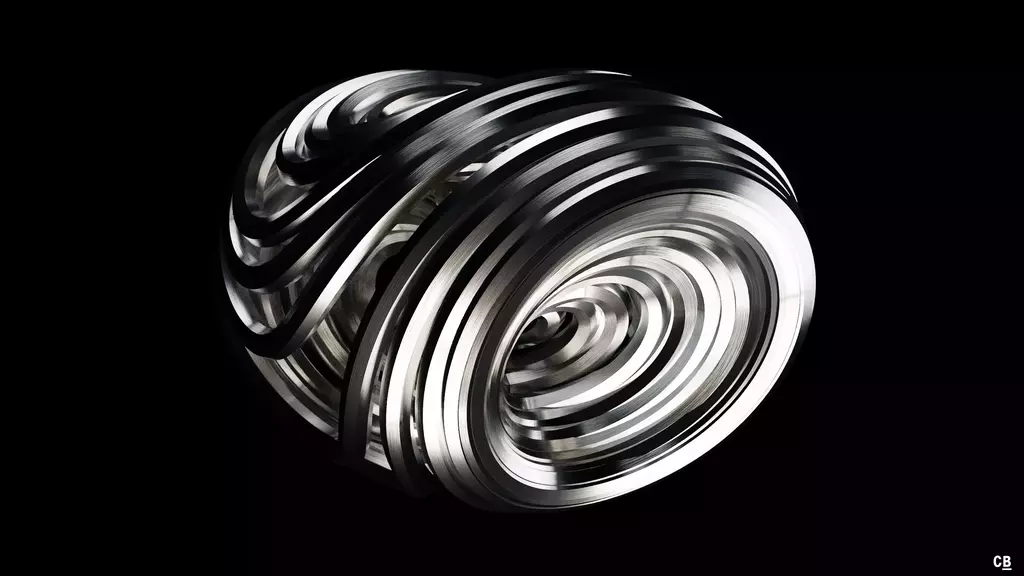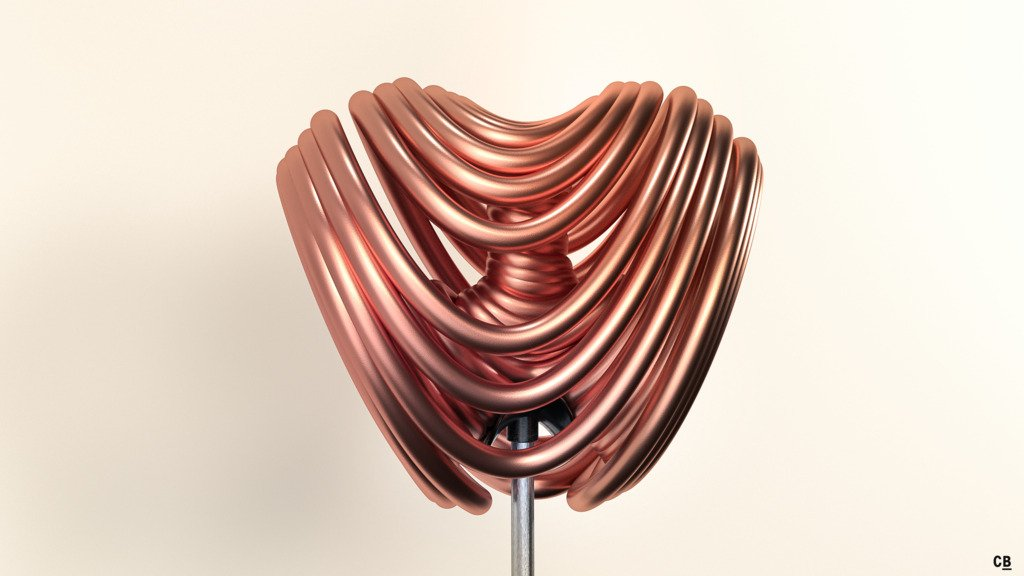# Li Attractor

This differential system found by Dequan Li in 2008 is the result of a research about the scrollness of attractors and is the first one with smooth quadratic terms that produces three scrolls1. The system is derived from the Lorenz system by generalizing it to a “fuzzy” version which was then simplified.

## Renders

Differential system:

$\dot{x} = a\, (y - x) + d\, x\, z$ $\dot{y} = k\, x + f\, y - x\, z$ $\dot{z} = - e\, x^2 + x\, y + c\, z$

Constants:

$a = 40$ $d = 0.16$ $k = 55$ $f = 20$ $e = 0.65$ $c = 11/6$## Stereographic Animation

1. D. Li, 2008. "A three-scroll chaotic attractor". Phys. Let. A. 372(4). doi:10.1016/j.physleta.2007.07.045

🕸 💍